## Aptitude :: Height And DistancePage 1

1.

 A ladder learning against a wall makes an angle of 60Â° with the ground. If the length of the ladder is 19 m, find the distance of the foot of the ladder from the wall. (a)9 m (b)9.5 m (c)10.5 m (d)12 m
Answer is: B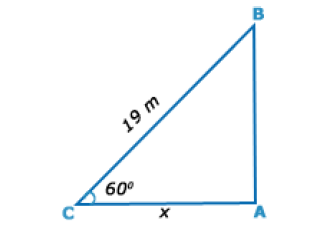Let AB be the wall and BC be the ladder.
Then, < ABC = 60Â°
and, BC = 19 m.;
AC = x metres
AC/BC = cos 60Â°
x / 19 = 1 / 2
x = 19/2
x = 9.5 m.

2.

 The angle of elevation of a ladder leaning against a wall is 60Â° and the foot of the ladder is 4.6 m away from the wall. The length of the ladder is ? (a)2.3 m (b)4.6 m (c)7.8 m (d)9.2 m
Answer is: DLet AB be the wall and BC be the ladder.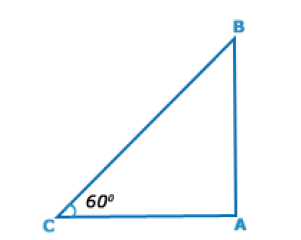Then, < ABC = 60Â°
AC = 4.6 m.;
AC/BC = cos 60Â° = 1 /2
BC = 2Ã—AC
BC = (2Ã— 4.6) m
BC = 9.2 m

3.

 The angle of elevation of the sun, when the length of the shadow of a tree is âˆš3 times the height of the tree is. (a)30Â° (b)45Â° (c)60Â° (d)90Â°
Answer is: ALet AB be the tree and AC be its shadow.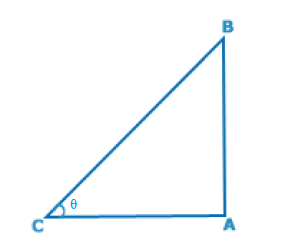Then, < ABC = Î¸.
then, AC/AB = âˆš3
Cot Î¸ = âˆš3 = cot 30Â°
Î¸ = 30Â°

4.

 If the height of a pole is 2âˆš3 meters and the length of its shadow is 2 meters, find the angle of elevation of the sun. (a)30Â° (b)45Â° (c)60Â° (d)90Â°
Answer is: CLet AB be the pole and AC be its shadow.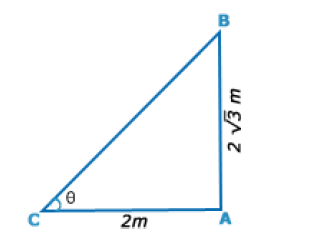Then, < ACB = Î¸.
Then, AB= 2 âˆš3 m, AC = 2m,
TanÎ¸ = AB/AC = 2âˆš3/2 = âˆš3
Î¸ = 60Â°

5.

 A man standing at a point P is watching the top of a tower, which makes an angle of elevation of 30Â° with the manâ€™s eye. The man walks some distance towards the tower to watch its top and the angle of the elevation becomes 60Â°. What is the distance between the base of the tower and the point P? (a)4âˆš3 units (b)8 units (c)12 units (d)Data inadequate

6.

 A man is walking along a straight road. He notices the top of a tower subtending an angle A = 60o with the ground at the point where he is standing. If the height of the tower is h = 30 m, then what is the distance (in meters) of the man from the tower? (a)15.23 (b)17.32 (c)20 (d)25.32
Answer is: B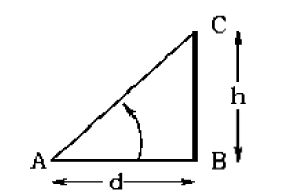Let BC represent the tower with height h = 30 m, and A represent the point where the man is standing. AB = d denotes the distance of the man from
tower.
The angle subtended by the tower is A = 60o
From trigonometry,
tan A = tan 60o = h / d = âˆš3
So d = 30/âˆš3 s m
Hence the distance of the man from the tower is 17.32 m

7.

 Two towers face each other separated by a distance d = 25 m. As seen from the top of the first tower, the angle of depression of the second tower's base is 60o and that of the top is 30o. What is the height (in meters) of the second tower? (a)28.87 (b)25.52 (c)25 (d)28
Answer is: A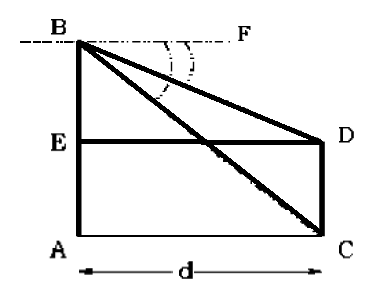The first tower AB and the second tower CD are depicted in the figure on the left.
First consider the triangle BAC. Angle C = 60o
tan BCA = tan 60o = AB/AC
This gives AB = d tan 60o
Similarly for the triangle BED, BE = d tan 30o
Now height of the second tower CD = AB âˆ’ BE = d (tan 60o âˆ’ tan 30o)
CD = 25 (âˆš3 âˆ’ 1/âˆš3) = 25 Ã— 2/âˆš3 = 28.87 m.

8.

 Two men on opposite sides of a TV tower of height 26 m notice the angle of elevation of the top of this tower to be 45o and 60o respectively. Find the distance (in meters) between the two men. (a)42 (b)41.01 (c)26 (d)45
Answer is: B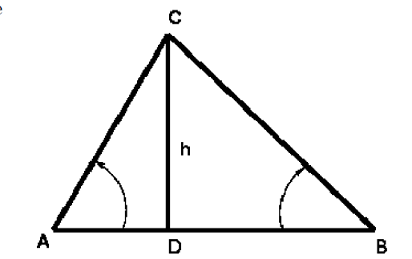The situation is depicted in the figure with CD representing the tower and AB being the distance between the two men.
For triangle ACD,
tan A = tan 60o = CD / AD
Similarly for triangle BCD,
tan B = tan 45o = CD/DB
The distance between the two men is
AB = AD + DB = (CD/tan 60o) + (CD/tan 45o)
AB = (26/âˆš3) + (26/1) = 41.01 m